## Hypersphere CosmologyAlternative to big-bang

Wednesday, 02 July 2014 15:29

## Hypersphere Visualisation & LensingThis paper provides a method of visualising the four dimensional hypersphere as a perspective construction in three dimensions, and it also shows how the curvature of hyperspherical space will distort images from distant galaxies and supernovae.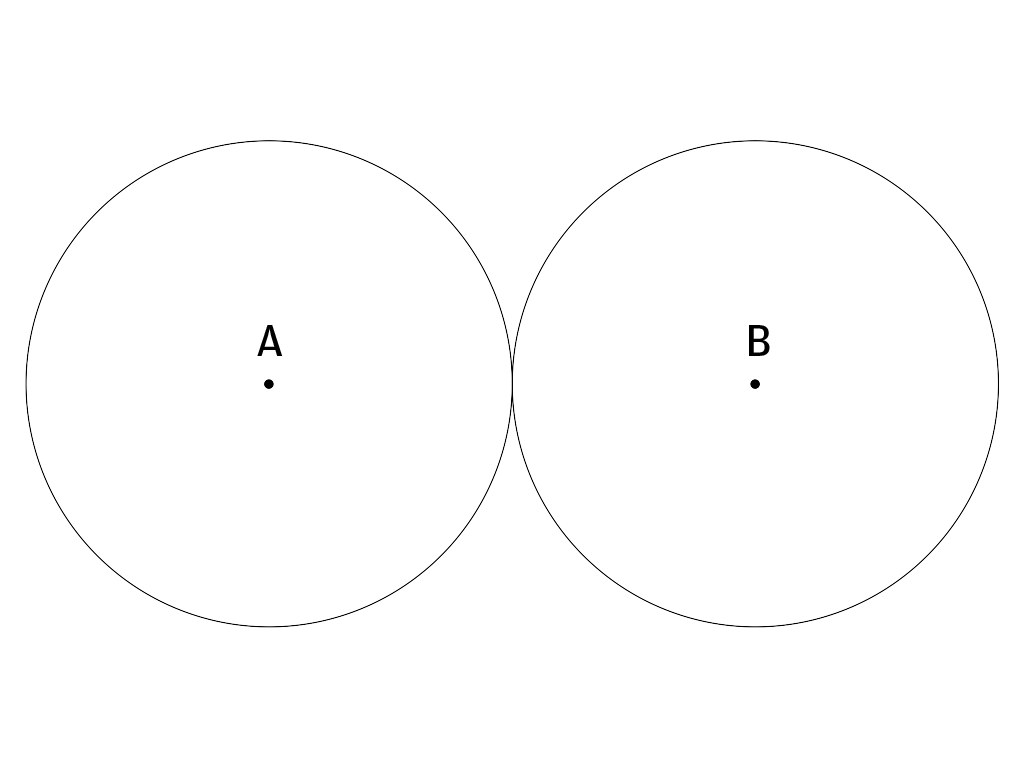fig 1

Figure 1. Any attempt to project the surface of the Earth onto a flat surface necessarily leads to some kind of distortion. In addition to the usual Mercator projection that distorts distances towards the poles, we can also make a polar projection by cutting through the equator and then "photographing" each hemisphere from above the poles. Such a projection gives a good representation of distances near the poles but it leads to progressive distortions as we near the equator. Cartographers normally place the two halves of the polar projection in contact at some point, to remind us that all points around the equator of one hemisphere actually touch a corresponding point on the other hemisphere.
Similarly we can represent the 3-sphere or hypersphere as two spheres in which every point on the surface of one of the spheres corresponds to a point on the other sphere, despite that we can only represent them with a single point of contact. Now when we make a polar projection of the Earth's surface, convention dictates that we centre the projection on the poles, but we could chose any two opposite points and cut the sphere across a great circle other than the equator. An egomaniac might delight in a projection with his house at the very centre of the projection, but it would remain a valid projection.
Thus an observer A, in an hypersphere can define her map of it with herself at the centre of one of the spheres. This then defines a second point B, as her hyperspherical antipode, analogous to the point furthest away from her on the surface of the world. In a hypersphere it represents the furthest point you can travel to without starting to come back to where you started from.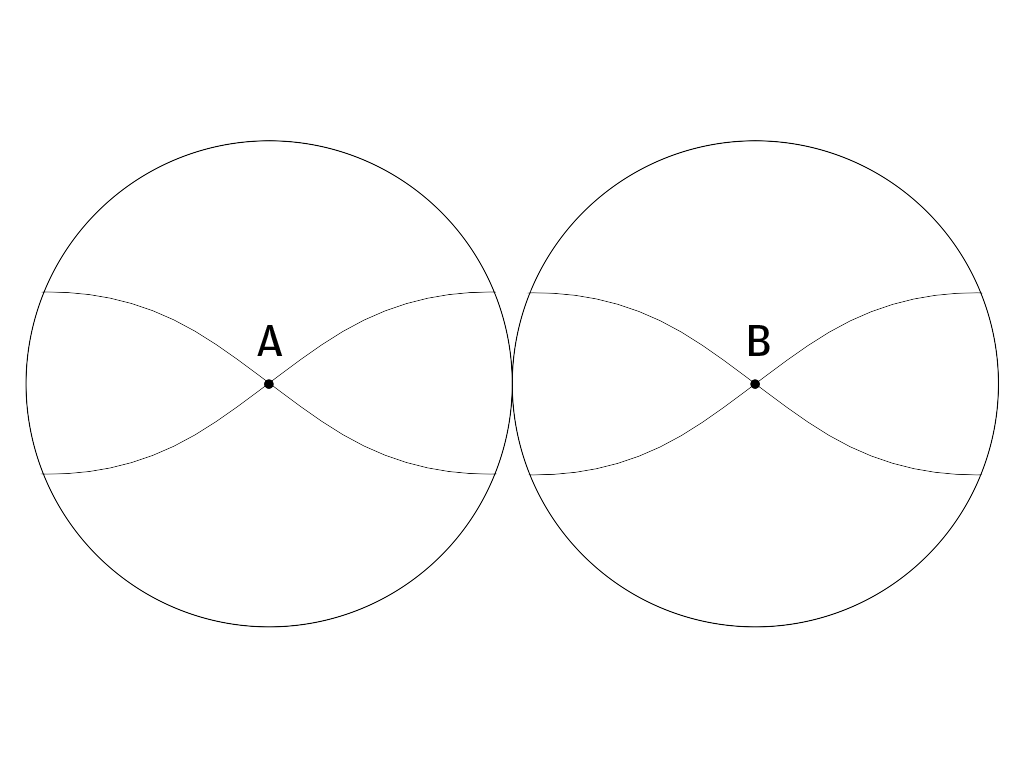fig2

Figure 2 . An observer looking into the deep space of an hypersphere could in theory see an abject at her antipode point B, by looking in any direction, analogous to the way in which all routes from the North Pole lead to the South Pole on the Earth. Note that lines of sight curve within an hypersphere, in a way analogous to the way in which meridians curve around the surface of the Earth. Light follows geodesics in space, so if space curves, light has to follow the curvature. Theoretically our observer could see right past her antipode and catch sight of the back of her own head In practice light from near the antipode becomes so red-shifted by the time it gets to A, that A cannot even see quite as far as the antipode.
VHC argues that the curvature of the universe also causes the progressive red-shift of light travelling across it, and that conventional cosmology has mistaken this for recession velocity, an hypothesis which implies an expanding universe. This paper will attempt to show that the supposed acceleration of that expansion arises from the lensing effect of hyperspherical space.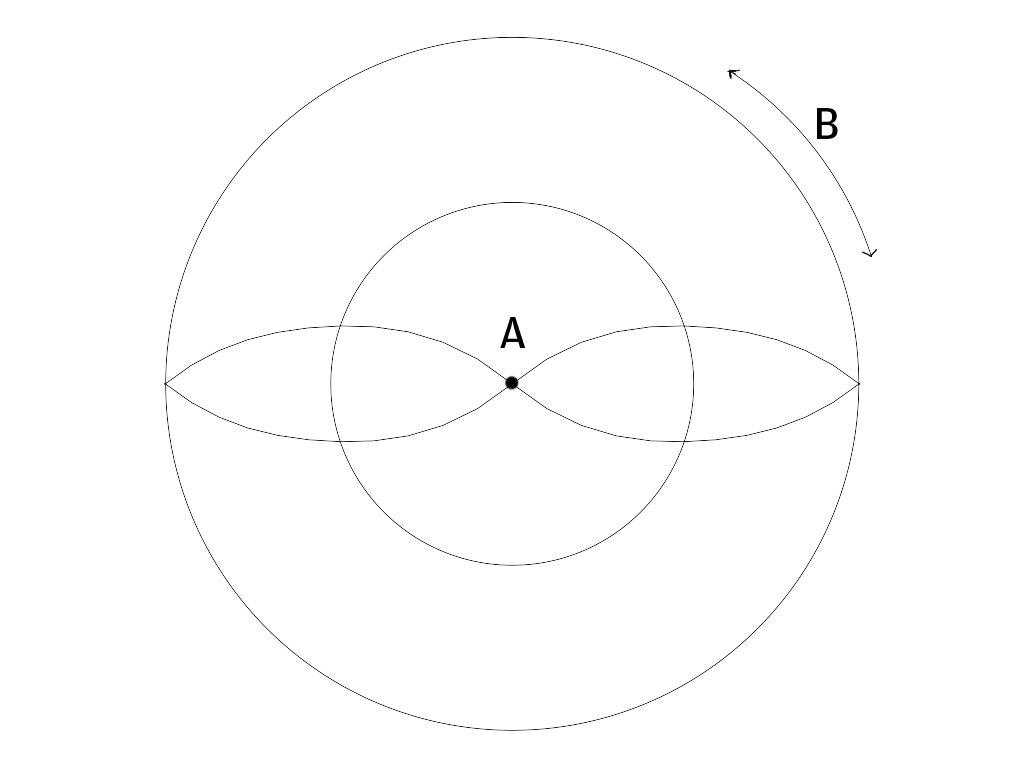fig3

Figure 3. Imagine that we take the polar projection of the Earth and then roll the equator of the Southern Hemisphere around that of the Northern one. This will have the effect of stretching out Antarctica so that it goes all the way around the circumference of the whole map. We would then have a circular and highly topological map of the world with huge distance distortions towards the South Pole which itself now stretches around the entire edge. With a little effort at visualisation we can do something analogous with the two sphere map of the hypersphere, by rolling one sphere all over the entire surface of the other so that all corresponding points come into contact. This will result in the antipode point becoming spread out over the entire surface of the resulting sphere. Astronomers who assume a "flat" Euclidean universe will have effectively and unwittingly distorted their view of the universe in exactly this way if it does in fact have an hyperspherical geometry.fig4

Figure 4. Astronomers who assume a flat universe with no curvature will assume that they can see in straight lines. If they look out into the apparent sphere of space that surrounds them, they will actually see along geodesics which curve relative to the assumed flat space of their maps.

The positive curvature of the Universe has a lensing effect on the passage of light across it: -=Where= hyperspherical lensing and d stands for the ratio a/L, astronomical distance over antipode distance. . The lensing distorts apparent magnitude and thus creates a mismatch with redshift leading to the dubious assumption of an expanding acceleration.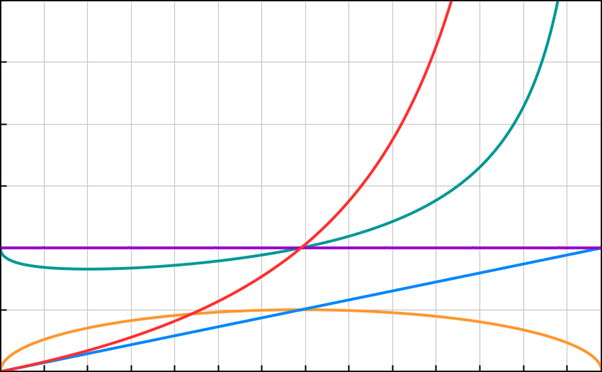The vertical axis for a number of factors runs from zero to three, marked in divisions of 0.5 with the unity line highlighted in purple for clarity. The horizontal axis runs from observer to antipode.

The red line shows redshift Z, where Z = (c/(c-(dA)^0.5)-1 where d = astronomical distance.

Note that a redshift of 1 at about 7 billion light years denotes the halfway point to the antipode distance. Redshift climbs exponentially towards infinity at the antipode; observations become increasingly difficult up to redshift 10 and then virtually impossible beyond.

The yellow line represents schematically the hyperspherical geodesic from the observer to the observer’s antipode; the curved path that light actually takes in the cosmic hypersphere.

The blue line represents schematically the observer’s assumed sight line for flat space.

The green line represents schematically the difference between the actual and the assumed sight line, and thus the degree of Hyperspherical Lensing LH, that light becomes subject to at various distances. Note that in this revised version of the model, the line has the inverse configuration to previous models on this site and the equation governing it has the form

LH  = 1/(1+((d-d^2)^0.5))-d) where here, d = astronomical distance/antipode distance.

The negative lensing at distances below 7 billion light years explains the anomalously low luminosity of type 1A supernovae without recourse to the hypothesis of an accelerating expansion driven by some mysterious dark energy.

The positive lensing at distances greater than 7 billion light years explains the increase in angular size of very distant structures without recourse to the hypothesis of an expanding universe at all.

Dark energy does not exist.

Thus the Universe consists of a hypersphere, finite but unbounded in both space and time and constant in size, and vorticitating with a small positive spacetime curvature, despite the apparent temporal and spatial horizons.

Inflation did not occur.

• #### HyperSphere Cosmology +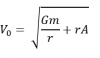Hypersphere Cosmology in a Nutshell   1a) The Universe consists of a Hypersphere (a 3-Sphere or 4-ball) where: -       also
• #### Hypersphere Redshift +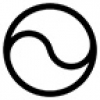The alternative mechanism for redshift works as follows: Redshift = Z =   - 1 Where = observed wavelength, = expected wavelength, and the -1
• #### Hypersphere Rotation +The Gödel 3d rotating universe has this solution derived from General Relativity: - “Matter everywhere rotates relative to the compass of
• #### Hypersphere Holometry - A Prediction +As a consequence of the hypothesis that the universe exists as a hypersphere where   the main parameters of the
• #### VHC. Vorticitating Hypersphere Cosmology +The overwhelming majority of university and state funded cosmologists believe that the observable universe has expanded from a much smaller
• #### Hypersphere Visualisation & Lensing +This paper provides a method of visualising the four dimensional hypersphere as a perspective construction in three dimensions, and it
• #### Sacred Geometry +

As faith began to make way for reason during the Enlightenment, humans began to deduce the relationships between certain mathematical
• #### Starships +

Houston we have a problem. If the human race does not develop starships that can reach other star systems as
• #### Quantum & Probability +

I have repeatedly asserted in many of my magical and scientific books and papers that this universe runs on probability
• #### Ur Chaos Theory +

In this paper 'Chaos' denotes randomness and indeterminacy, not the mere so-called 'deterministic chaos' of systems with extreme sensitivity to
• 1